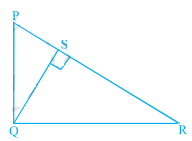" ">

# In figure below, $\mathrm{PQR}$ is a right triangle right angled at $\mathrm{Q}$ and $\mathrm{QS} \perp \mathrm{PR}$. If $P Q=6 \mathrm{~cm}$ and $P S=4 \mathrm{~cm}$, find $Q S, R S$ and $Q R$."

Given:

$\mathrm{PQR}$ is a right triangle right angled at $\mathrm{Q}$ and $\mathrm{QS} \perp \mathrm{PR}$.

$P Q=6 \mathrm{~cm}$ and $P S=4 \mathrm{~cm}$.

To do:

We have to find $Q S, R S$ and $Q R$.

Solution:

In $\triangle S Q \mathrm{P}$ and $\triangle \mathrm{SRQ}$,

$\angle P S Q =\angle R S Q=90^o$

$\angle S P Q =\angle S Q R=90^{\circ}-\angle R$

Therefore, by AA similarity,

$\Delta S Q P \sim \Delta S R Q$

This implies,

$\frac{S Q}{P S}=\frac{S R}{SQ}$

$S Q^{2}=P S \times S R$.......(i)

In right angled triangle $P S Q$, by Pythagoras theorem,

$P Q^{2} =P S^{2}+Q S^{2}$

$(6)^{2}=(4)^{2}+Q S^{2}$

$36 =16+Q S^{2}$

$Q S^{2}=36-16$

$=20$

$QS=\sqrt{20}$

$=2 \sqrt{5} \mathrm{~cm}$ Substituting the value of $Q S$ in (i), we get,

$(2 \sqrt{5})^{2}=4 \times S R$

$S R =\frac{4 \times 5}{4}$

$=5 \mathrm{~cm}$

In right angled triangle $Q S R$,

$Q R^{2} =Q S^{2}+S R^{2}$

$Q R^{2}=(2 \sqrt{5})^{2}+(5)^{2}$

$Q R^{2}=20+25$

$Q R=\sqrt{45}$

$=3 \sqrt{5} \mathrm{~cm}$

Hence, $Q S=2 \sqrt{5} \mathrm{~cm}, R S=5 \mathrm{~cm}$ and $Q R=3 \sqrt{5} \mathrm{~cm}$

Updated on: 10-Oct-2022

28 Views